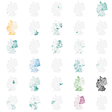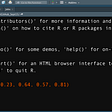# Create LaTeX Tables in R

## A Step by Step Tutorial

In this article, we will discuss how to create tables in R using the KableExtra package. Although our main focus is to create a table in LaTex format, in case you prefer writing documents in MS Word, we will also show how to manually export KableExtra tables to MS Word.

For this lesson, we are using the Financial Well-Being Survey 2016 data. This survey was conducted by the Consumer Financial Protection Bureau (CFPB) and the data is publicly available here (https://www.consumerfinance.gov/data-research/financial-well-being-survey-data/). In our table, we want to show the descriptive statistics of the Financial Well-Being Score (FWBScore variable) by gender (PPGENDER variable) and educational attainment (PPEDUC variable).

`data <- read.csv("https://www.consumerfinance.gov/documents/5614/NFWBS_PUF_2016_data.csv")`

Now, we install (assuming we did not install them before) and load the dplyr and the KableExtra packages:

`install.packages("dplyr")install.packages("kableExtra")library(dplyr)library(kableExtra)`

Now that we are all set, we will follow these steps:

1. Using the group_by and summarise functions of the dplyr package, we will prepare the descriptive statistics that we want to show in the table. So, at first, we create a dataframe with descriptive statistics.
2. Next, we pass this dataframe inside the kbl function of the kableExtra package.
3. Lastly, we add a caption to the table and select fonts and alignment.

In this dataset, the PPGENDER==1 is male and PPGENDER ==2 is female; and, PPEDUC==1 is less than high school, PPEDUC ==2 is high school degree/GED, PPEDUC ==3 is some college/associate, PPEDUC==4 is bachelor’s degree, and PPEDUC==5 is graduate/professional degree. To help our readers better understand the table, before creating it, we should recode the levels of the two variables.

`data\$PPGENDER <- recode_factor(data\$PPGENDER, "1"="Male",                                              "2"="Female")data\$PPEDUC <- recode_factor(data\$PPEDUC,                             "1"="less than high school",                            "2"="high school degree/GED",                            "3"="some college/associate",                            "4"="bachelor's degree",                            "5"="graduate/professional degree")`

Let’s create a summary statistics (count of observations, mean, median, and standard deviation) of financial well-being score by gender and education and put the output inside a dataframe called “table”:

`table <- data %>% group_by(PPGENDER,PPEDUC) %>%  summarise(Count=n(),            Mean.FWBScore=mean(FWBscore),            Median.FWBScore=median(FWBscore),            SD.FWBScore=sd(FWBscore)            )table`

The dataframe looks okay, but we do not want these many digits after the decimal. Let’s fix this by adding the round function in the previous code to select only one digit after the decimal:

`table <- data %>% group_by(PPGENDER,PPEDUC) %>%  summarise(Count=n(),            Mean.FWBScore=round(mean(FWBscore),digits=1),            Median.FWBScore=round(median(FWBscore),digits=1),            SD.FWBScore=round(sd(FWBscore),digits=1)  )`

Great! Now, we will convert this dataframe into a HTML table:

`table %>%  kbl(caption="Table 1: Summary Statistics of Financial Well-Being                 Score by Gender and Education",       format= "html",   col.names = c("Gender","Education","Count","Mean","Median","SD"),                  align="r") %>%   kable_classic(full_width = F, html_font = "helvetica")`

In the above code, we can change alignment by changing the value of align (“r”=right, “l”=left, “c”=center). Also, we can change the font type by changing the value of html_font (e.g., “Arial Narrow”, “Trebuchet MS”, “Source Sans Pro”, etc.). And, in case we would like to change the table style, in place of kable_classic, we can try: Kable_classic_2, Kable_minimal, Kable_material, Kable_material, Kable_material_dark, and kable_paper.

For example, if we try kable_minimal, this is what we get:

Okay! Now it is time to convert this table into LaTeX format. We just change format = “html” to format=“latex”. Also, we do not include “Table 1” in the caption (like we did in case of the HTML table) because LaTeX editors automatically generate a table number.

`table %>%  kbl(caption="Summary Statistics of Financial Well-Being Score by Gender and Education",      format="latex",      col.names = c("Gender","Education","Count","Mean","Median","SD"),      align="r") %>%  kable_minimal(full_width = F,  html_font = "Source Sans Pro")`

Once we run the code, in R, we see the following output:

This is the LaTeX code that we need to paste into any LaTeX editor to create the table. I paste the code into Overleaf (https://www.overleaf.com/) and this is what I get:

Now, if you are a loyal MS Word user and would like to have this table in a word document, do the following:

1. In Viewer, click Zoom.

2. Once you are in Viewer Zoom, press ctrl + A to select everything:

3. Paste the table in your MS Word document:

Now, this requires some editing!

4. Select the table.

5. Click: Insert -> Table -> Insert Table:

Now, the table looks like this:

Finally, I do some more easy editing in MS Word and get the following table:

Thanks for following along! In case you are interested in more descriptive statistics lessons, have a look at some of my previous articles:

--

--

--

## More from Vivekananda Das

Sharing ideas on Cause-and-Effect, Data Analysis in R, Stat Literacy, and Happiness | Ph.D. student @UW-Madison | More: https://vivekanandadas.com

Love podcasts or audiobooks? Learn on the go with our new app.

## Basic Inferential Statistics for Data Science programmers-I## R Weekly 2017–41 Raspberry Pi, Markdown## I Used Data Analytics To Figure Out How To Rank High On Medium## Two ways to construct Ridge regression.## Answering the ‘What If” Questions — CFOs and Scenario Modeling## The Eye of the Storm## Finding Similar Names using Cosine Similarity## Analytics Systems Have Been Democratized. We Still Need Curious Business Minds to Drive Forward.## Vivekananda Das

Sharing ideas on Cause-and-Effect, Data Analysis in R, Stat Literacy, and Happiness | Ph.D. student @UW-Madison | More: https://vivekanandadas.com

## Basic functions that every R programmer should know about.## Writing Faster R with Vectorization and the {apply} family## How Vectors Influence When To Use For While Loops in R programming## R function of the week: Getting more out of the read.table() function